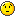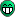# Fitting a Poisson Distribution to a histogram

Hi everyone and thank you for taking the time to read this postI have desperately been trying to fit a Poisson distribution with 3 parameters to a histogram.
Here is a very simple code which reflects my problem:

`````` {
TRandom *rand = new TRandom(0);
TH1F *hPois = new TH1F("hPois","hPois",100,0,100);
float val;
float lambda = 1.1;
for(int i=0;i<10000;i++){
val = (float)rand->Poisson(lambda);
hPois->Fill(val);
}
gROOT->SetStyle("Plain");
TCanvas *c = new TCanvas("c","c",800,600);
hPois->Draw();
TF1 *f1 = new TF1("f1","*TMath::Power((/),(x/))*(TMath::Exp(-(/)))/TMath::Gamma((x/)+1)",0,.1);
hPois->Fit("f1","R");
}
``````

I don’t manage to get anything out of the fit. The parameters are all 0 and will not change:

``````FCN=0 FROM MIGRAD    STATUS=CONVERGED     119 CALLS         120 TOTAL
EDM=0    STRATEGY= 1  ERROR MATRIX UNCERTAINTY 100.0 per cent
EXT PARAMETER                APPROXIMATE        STEP         FIRST
NO.   NAME      VALUE            ERROR          SIZE      DERIVATIVE
1  p0           0.00000e+00   1.41421e+00   0.00000e+00   0.00000e+00
2  p1           0.00000e+00   1.41421e+00   0.00000e+00   0.00000e+00
3  p2           0.00000e+00   1.41421e+00   0.00000e+00   0.00000e+00
``````

I have been wondering if the problem could come from the use of TMath in the TF1 but I don’t see how I can use a gamma function otherwise than by using TMathAny help would be greatly appreciated!Thanks!

Cecilia

``````TF1 *f1 = new TF1("f1","*TMath::Power((/),(x/))*(TMath::Exp(-(/)))/TMath::Gamma((x/)+1.)", 0, 10); // "xmin" = 0, "xmax" = 10
f1->SetParameters(1, 1, 1); // you MUST set non-zero initial values for parameters
hPois->Fit("f1", "R"); // "R" = fit between "xmin" and "xmax" of the "f1"
``````

I had tried that and it did not work… Now I tried it on another computer and it works. So I think that the global installation of root at the university is defective! Anyway this is embarassingBut thank you so much for your helpThis is a nice functionit fits my data a lot better than the standard poisson distributions i was trying to use. please could you explain how you take the mean and width from a distribution fitted with this function? It seems that the parameters don’t relate to the mean and width in the same way as for the standard poisson distribution.

cheersThe function you provided was extremely useful.

Thank you!

Hi All,

Could you please let me know how we can extract the mean and error on mean (sigma) from the function described in this fit?

``````TF1 *f1 = new TF1("f1","*TMath::Power((/),(x/))*(TMath::Exp(-(/)))/TMath::Gamma((x/)+1)", 0, 10);
``````

As per definition, if we compare it with

``````f(x) = ( u^x * e^{-u} )/ factorial(x)
``````

Where:

``````         = Normalizing parameter
 /  -> mean (mu)
x /  -> x
Gamma( x /  + 1 ) = factorial (x / )
``````

So, If you look at the attached fit from this function it fits very well. And the values of parameters are following:

par 0 = 35110
par 1 = 2.478
par 2 = 0.2349

So:

mean = par 1 / par 2 = 2.478/0.2349 = 10.55

But, the histogram mean is showing 2.09. The two values from fit and after fit seems very far. So, I am confused. Is this the actual mean or should we need to do something else to get mean?

with regards,
Ram

I guess, the trick with the parameter “” is to scale “x” and then also “u” (as they appear in your “definition”), so that the “mean” (and thus also the “variance”) remains simply the value of the parameter “”.

You could try to “scale” the “x-axis” of your histogram by the value of the parameter “” (then also possibly “`f1->FixParameter(2, 1.0);`”) and then redo your fit:

``````{
gStyle->SetOptFit(112);
Double_t xmin = 0, xmax = 10;
// define the Poisson fit function
TF1 *f = new TF1("f", "*TMath::Power((/),(x/))*(TMath::Exp(-(/)))/TMath::Gamma((x/)+1.)", xmin, xmax);
f->SetParameters(1, 1, 1); // you MUST set non-zero initial values for parameters
// define and "fill" the histogram
TH1D *h = new TH1D("h", "h", 10, xmin, xmax);
h->SetBinContent(1, 0);
h->SetBinContent(2, 2100);
h->SetBinContent(3, 4400);
h->SetBinContent(4, 1500);
h->SetBinContent(5, 200);
h->SetBinContent(6, 100);
h->SetBinContent(7, 50);
h->SetBinContent(8, 50);
h->SetBinContent(9, 20);
h->SetBinContent(10, 0);
// fit the histogram
h->Fit(f, "R"); // "R" = fit between "xmin" and "xmax" of the "f"
std::cout << "original mean = " << f->GetParameter(1)
<< " +- " << f->GetParError(1) << std::endl;
// return; // ... "break" here ...
// "scale" the "fix bins x-axis" of the histogram
Double_t s = f->GetParameter(2); // the fitted "scaling" parameter
xmin /= s; xmax /= s;
h->GetXaxis()->Set(h->GetNbinsX(), xmin, xmax);
h->SetTitle("h scaled");
// fit the "scaled" histogram
f->SetRange(xmin, xmax); // set the new "scaled" range
// f->FixParameter(2, 1.0); // fix the "scaling" parameter
h->Fit(f, "R"); // "R" = fit between "xmin" and "xmax" of the "f"
std::cout << "scaled mean = " << (s * f->GetParameter(1))
<< " +- " << (s * f->GetParError(1)) << std::endl;
}
``````

Thank you very much Pepe. Now I got it.

I don’t understand the mathematical legitimation of this manipulation. The introduction of an additional degree of freedom surely improves the goodness of fit, but how can we justify it? If the underlying distribution of the data is really Poissonian, then I don’t see how we are allowed to stretch the axis.
Cheers

It’s physics.1 Like